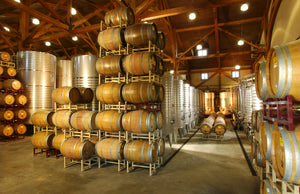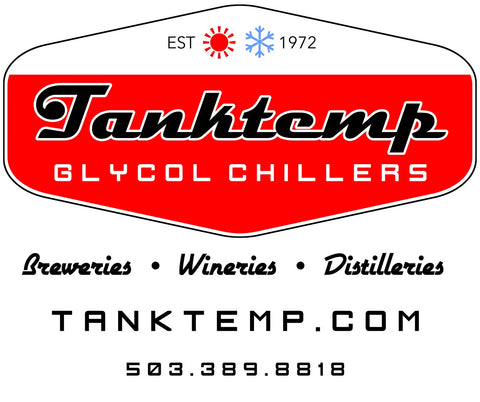Chiller sizing worksheet

January 22, 2019Project work sheet  ____________ Contact___________Phone__________ Email_______________

Size of tanks/ number of tanks   _____/_____    _____/_____    _____/_____    _____/_____

Temperature Differential Start   ____F   Finish____F  =____DT    Time to achieve temp________

Calculate the Pulldown load

1. Calculate the volume in gallons of your tanks (1bbl = 31 gallons) ex: 7bbl tank = 217 gallons per tank. Wine tanks are in gallons or liters vs

Assumption, we have four, 7bbl or 217 gallons per tank. 217 gallons x 4 tanks = 868 gallons

• Multiply the total gallons by 8.33 to get the total pounds
868 x 8.33 = 7230.44 pounds
• Multiply the total pounds by your temperature difference
Say 75°F – 34°F = 41°F
44 x 41 = 296,448 Total BTU
• Divide by the hours of your pull down. 24 hours
ex: 296,448/24 hrs = 12,352 (BTU/HR).  This is the BTU/HR required crash time process.  We suggest adding 10-15% to this number to accommodate for heat loss and thermal factors.  = 13,387 is the need BTU for this task in this time frame.

Add “Heat Load” for Active Fermentation we allow 280BTU (wine beer)

1. Multiply the total bbls (31gallons per barrels) by 15 brix. Or .5 Brix per gallon
ex: 6bbl x 15 = 90 brix
2. Multiply by 280 BTU
ex: 90 brix x 280 BTU = 25,200 BTU
3. Divide by 70 hours. 70 hours is the typically the amount of time that the majority of heat gain from yeast occurs, even if the fermentation period is longer than 75 hours.
ex: 25,200/70 = 360 BTU/HRNeeded for active fermentation

Add the “pulldown load” and the “Active fermentation load” together for the total BTU/HR load

ex:  12,352 (BTU/HR) + 360 BTU/HR = 12,712 BTU/HR is needed.

It is critical that the chiller you are purchasing is sized correctly, better to have more then you need then not enough.  Tanktemp Control Chillers are sized by horsepower not tonnage.  A machine is only as good as the parts it is made out of the craftsmanship of the assembly of those parts.

We are “Always over-built. Never over-engineered.”

Get in on thisJoin our low-volume mailing list (sent about once a month) and get news of our best deals first.  We respect your privacy and only email with permission. We never sell or share your info.  Ever.